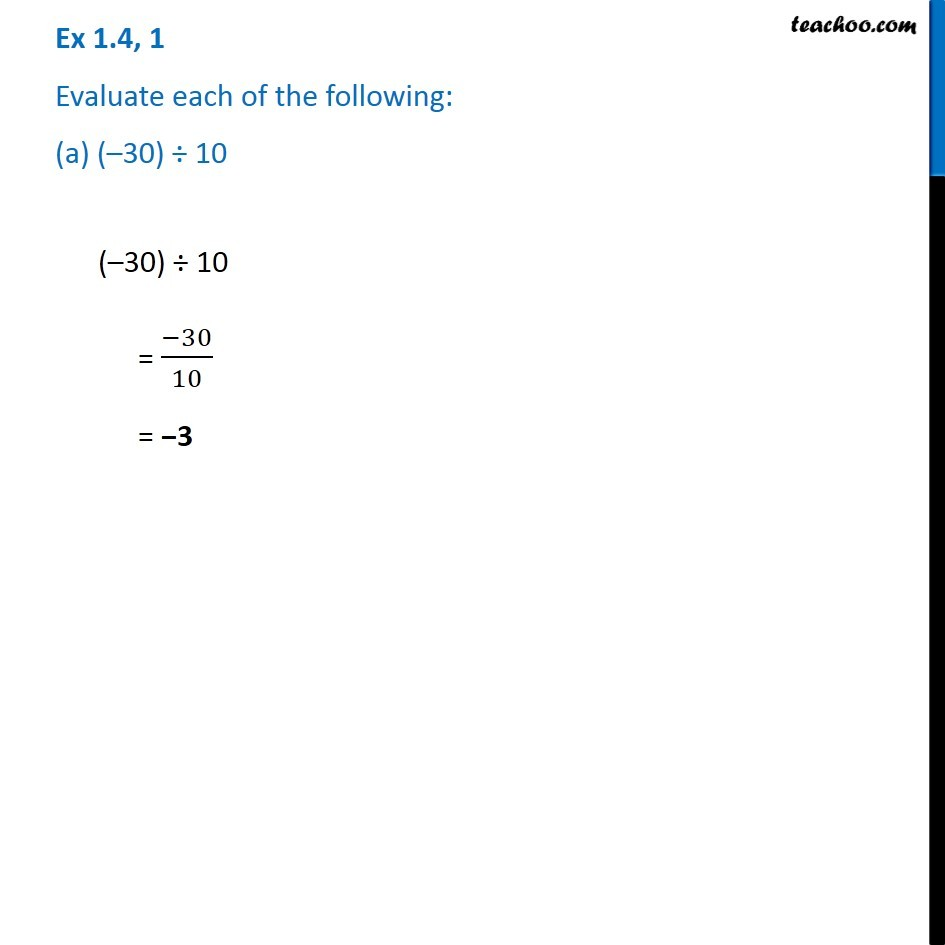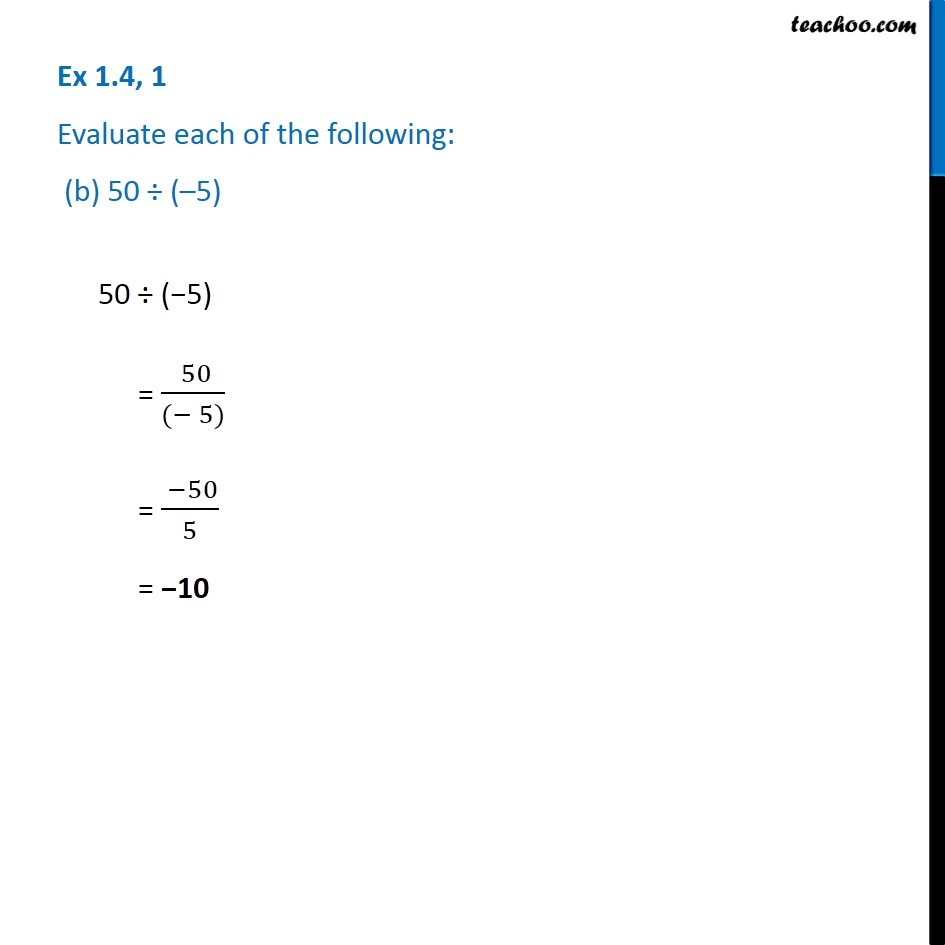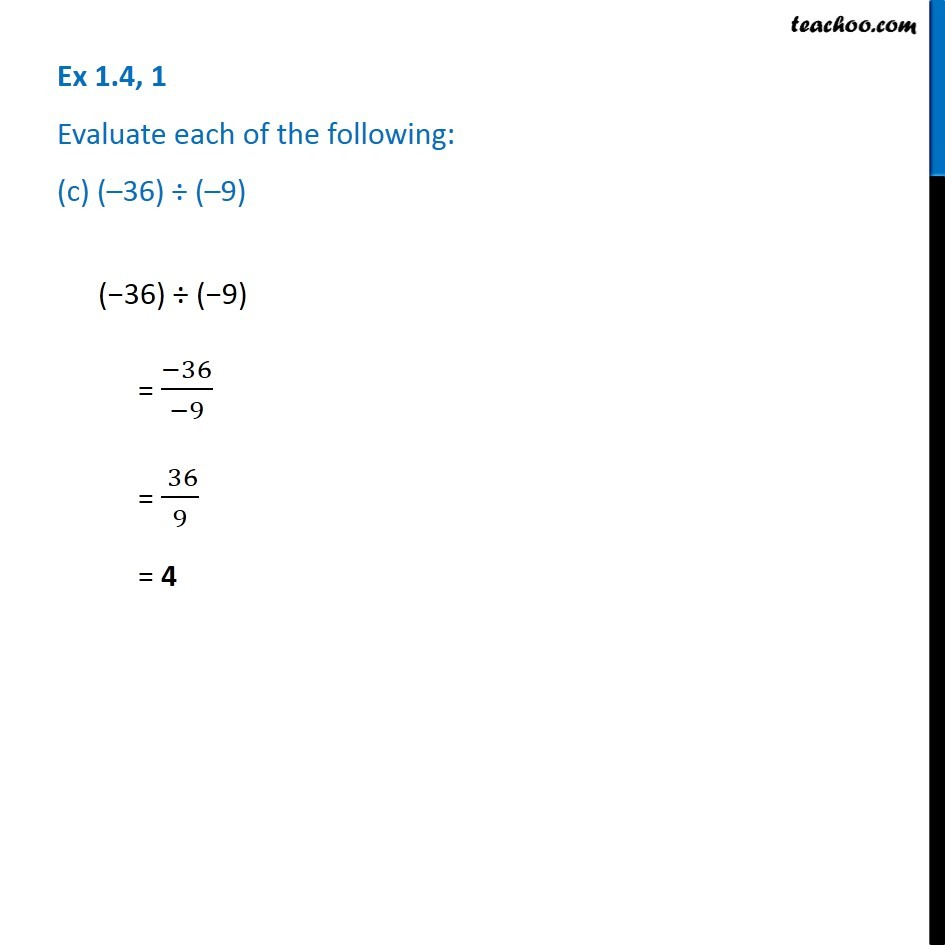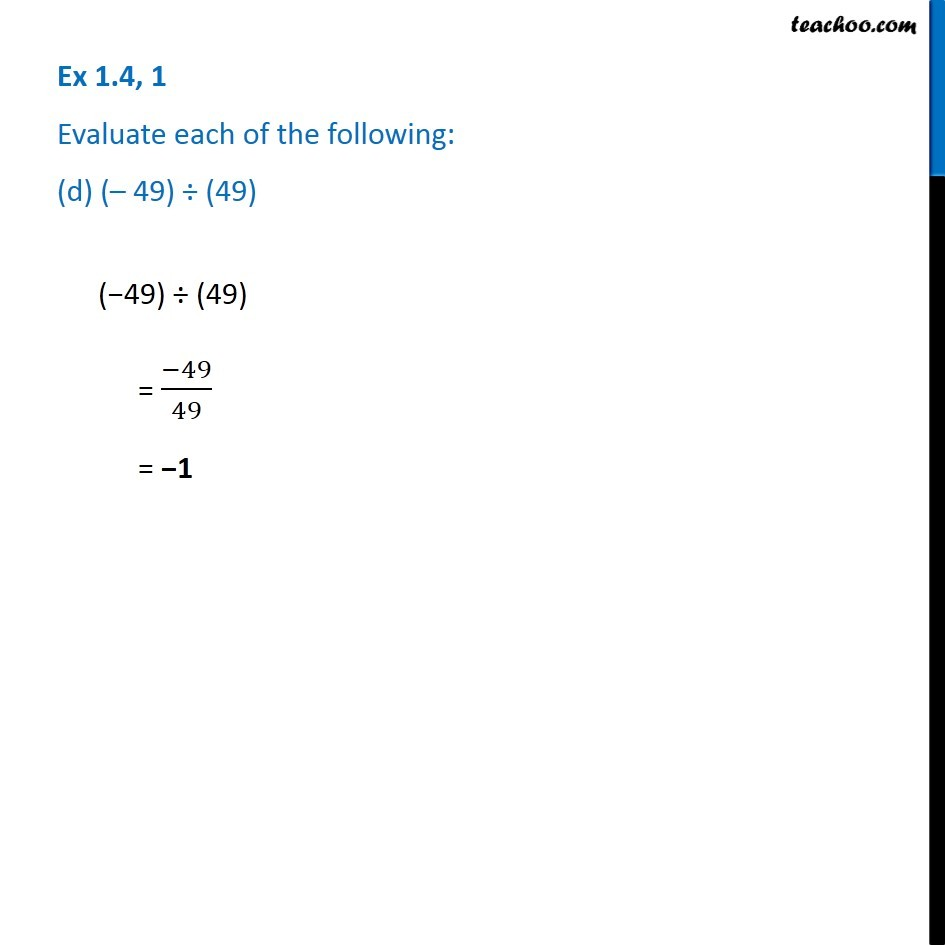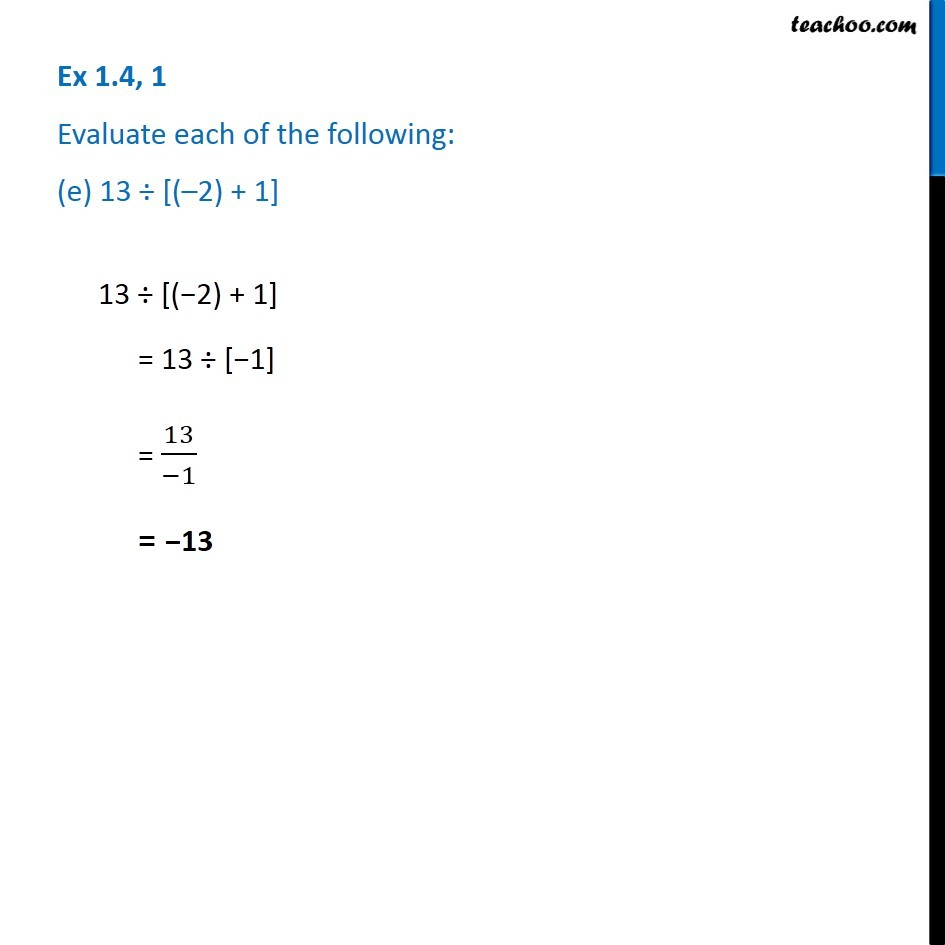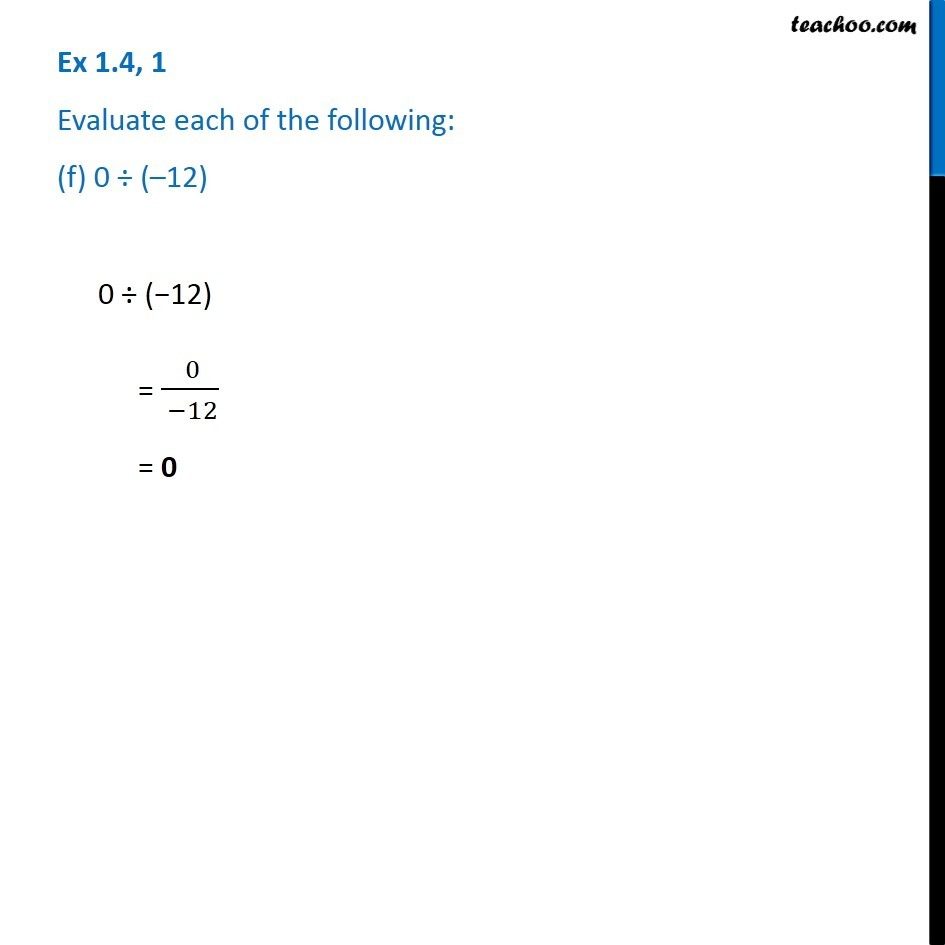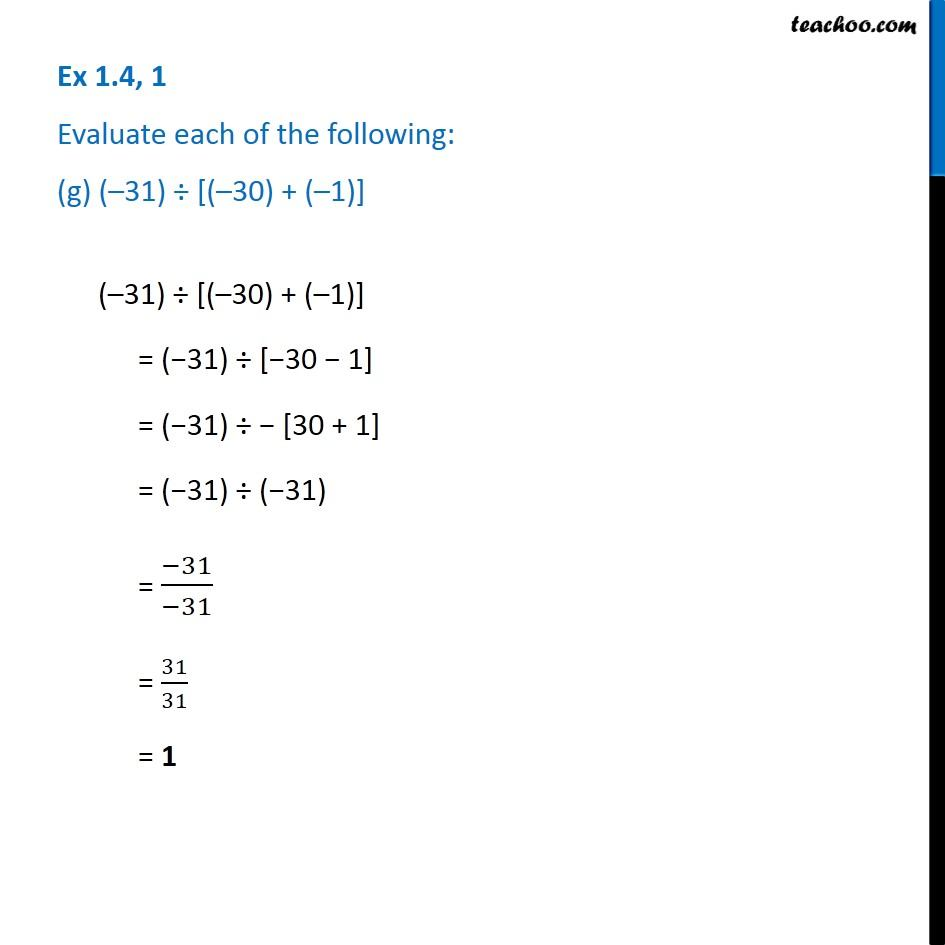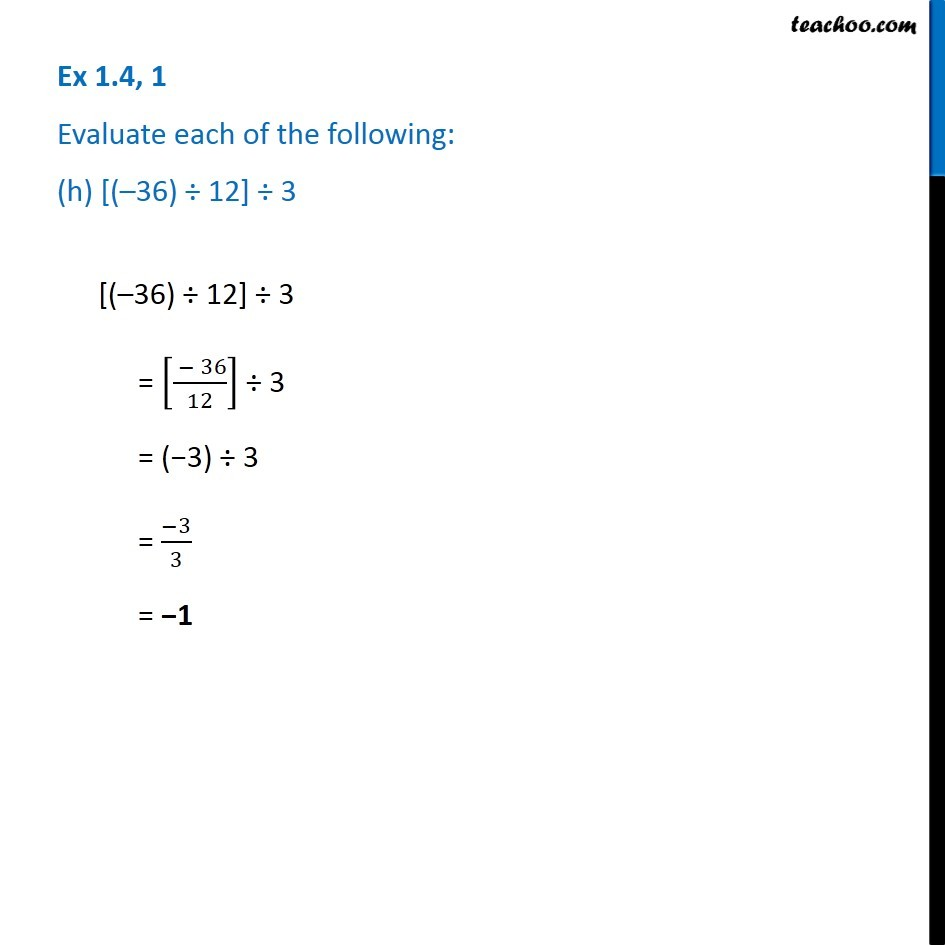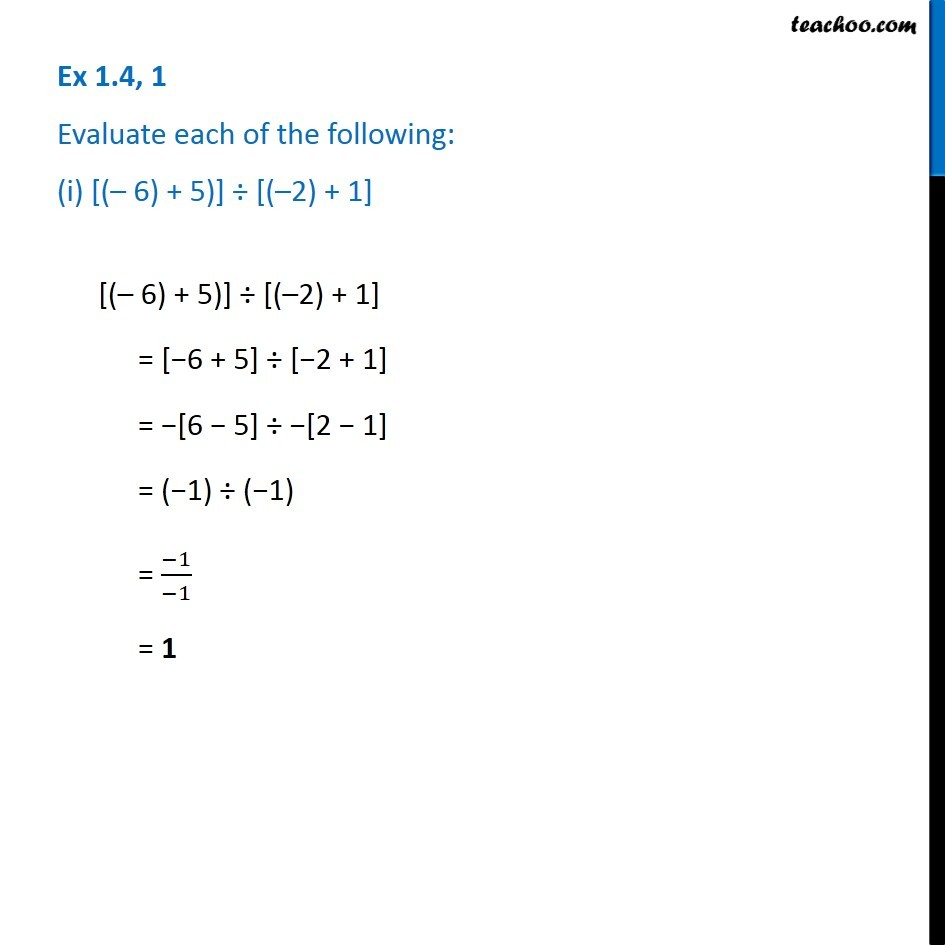Subscribe to our Youtube Channel - https://you.tube/teachoo

1. Chapter 1 Class 7 Integers
2. Serial order wise
3. Ex 1.4

Transcript

Ex 1.4, 1 Evaluate each of the following: (a) ( 30) 10 ( 30) 10 = ( 30)/10 = 3 Ex 1.4, 1 Evaluate each of the following: (b) 50 ( 5) 50 ( 5) = ( 50)/(( 5)) = ( 50)/5 = 10 Ex 1.4, 1 Evaluate each of the following: (c) ( 36) ( 9) ( 36) ( 9) = ( 36)/( 9) = ( 36)/9 = 4 Ex 1.4, 1 Evaluate each of the following: (d) ( 49) (49) ( 49) (49) = ( 49)/49 = 1 Ex 1.4, 1 Evaluate each of the following: (e) 13 [( 2) + 1] 13 [( 2) + 1] = 13 [ 1] = 13/( 1) = 13 Ex 1.4, 1 Evaluate each of the following: (f) 0 ( 12) 0 ( 12) = ( 0)/( 12) = 0 Ex 1.4, 1 Evaluate each of the following: (g) ( 31) [( 30) + ( 1)] ( 31) [( 30) + ( 1)] = ( 31) [ 30 1] = ( 31) [30 + 1] = ( 31) ( 31) = ( 31)/( 31) = 31/31 = 1 Ex 1.4, 1 Evaluate each of the following: (h) [( 36) 12] 3 [( 36) 12] 3 = [( 36)/12] 3 = ( 3) 3 = ( 3)/3 = 1 Ex 1.4, 1 Evaluate each of the following: (i) [( 6) + 5)] [( 2) + 1] [( 6) + 5)] [( 2) + 1] = [ 6 + 5] [ 2 + 1] = [6 5] [2 1] = ( 1) ( 1) = ( 1)/( 1) = 1

Ex 1.4

Chapter 1 Class 7 Integers
Serial order wise